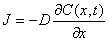# Fick's First Law of Diffusion-------------(1)

Where J is the rate of transfer of solute per unit area or the diffusion flux.

C is the concentration of solute

x is the co-ordinate axis in the direction of the solute flow.

t is the diffusion time

D is diffusivity

·         Equation (1) is called Fick's first law of diffusion.

·         It states that the local rate of transfer of solute per unit area per unit time is proportional to the concentration gradient of the solute, and defines the proportionality constant as the diffusivity of the solute.

·         The negative sign on the right hand side of the Equation (1) states that the matter flows in the direction of decreasing solute concentration i.e. gradient is negative.